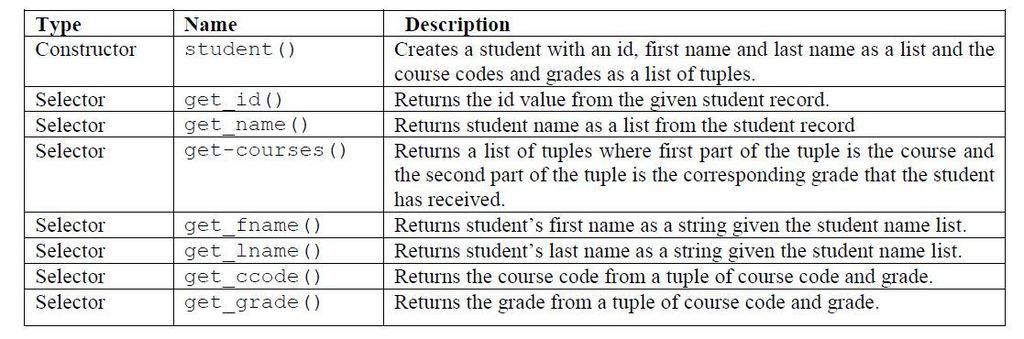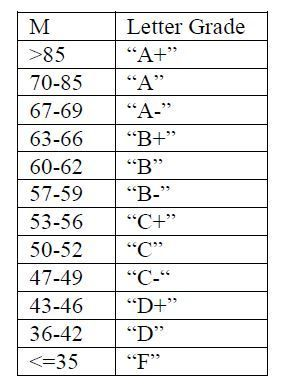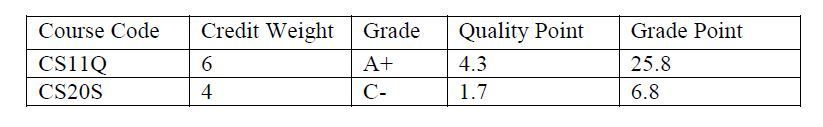Student Management System in PythonPosted by Samath20183 March 25, 2015

A student record contains the following information: id, name, list of courses with a corresponding grade. A name consists of a first name and a last name. The following functions have been provided:Write a function calc_letter_grade() which takes a student as input and returns a list of tuples where the first part of the tuple is the course code and second part of the tuple is the letter grade.
From the student structure, first extract the course list which is a list of tuples of course codes and the number grades. Get the number grades from the course list and create a new list in which each number grade is converted to a letter grade. Recreate a new courses list with the list of courses and the list of letter grades.

{Hint: Use map to apply a function to every element of the list. Also remember that zip takes two lists as inputs and creates a list of tuples e.g. zip ([1,2,3] ,[4,5,6]) => [(1, 4), (2, 5), (3, 6)] }
[('cs11q','A'),('cs11r','B'),('cs20r','C'),('cs20s','B'),('cs22q','B+'),('cs23q', 'A')]

To calculate Grade point average (GPA) the letter grade for each course and the quality points for each letter grade are required. Each course has a corresponding credit weight associated with it and each letter grade has a corresponding quality point associated with it.
For each course a student has taken a grade point is calculated by multiplying the quality point associated with the letter grade that the student has gotten by the credit weight for that course. The GPA is calculated by dividing the total grade points of all courses by the total amount of credit hours.
For example if a student has taken two courses CS11Q and CS20S and the grades are “A+” and “C-”, then the grade point average would be calculated as follows;GPA = (25.8+6.8)/10 = 3.26

Write a function convert_to_wtqp() which takes a tuple of course code and the letter grade and uses these as keys to get the corresponding values from the dictionaries credit_list and qp_list and returns the values as a tuple. To access the first part of the tuple use the selector function get_ccode() and the second part of the tuple use get_grade()
>>> convert_to_wtqp(("cs11q","A+"))
(6, 4.3)

Write a function calc_gpa() which takes a student record and calculates the gpa for the student. First extract the list of tuples of course codes and number grades and create a list of tuples with course codes and letter grade. Then create a list of corresponding wt and qp for each course code and letter grade, this can be created by applying convert_to_wtqp() to each element of the course code and letter grade list. Accumulate grade_points and credit_weights as shown in the table above and divide total grade points by total credit weights to calculate the Grade Point Average (GPA).
Use the function print_students_gpa(),which takes a student record as a parameter, to print the student’s GPA.

Code:

```def student(sid,fname,lname, cc1,mark1,cc2,mark2,cc3,mark3,cc4,mark4,cc5,mark5,cc6,mark6):
"""Constructor for student"""
return [sid,[fname,lname],[(cc1,mark1),(cc2,mark2),(cc3,mark3),(cc4,mark4),(cc5,mark5),(cc6,mark6)]]

def get_id(std):
"""Returns students ID"""
return std

def get_name(std):
"""Returns students Name"""
return std

def get_courses(std):
"""Returns a list of tuples of course codes and grade"""
return std

def get_fname(name):
"""Returns first name"""
return name

def get_lname(name):
"""Returns last name"""
return name

def get_ccode(course_det):
"""Returns course code part of the tuple"""
return course_det

"""Returns grade part of the tuple"""
return course_det

st1=student("620000101","John","Doe","CS11Q",80,"CS11R",60,"CS20R",50,"CS20S",60,"CS22Q",65,"CS23Q",80)

credit_list={'CS11Q':6,'CS11R':6,'CS20R':4,'CS23Q':4,'CS22Q':4,'CS20S':4}

qp_list = {"A+":4.3,"A":4.0,"A-":3.7,"B+":3.3,"B":3.0,"B-":2.7,"C+":2.3,"C":2.0,"C-":1.7,"D+":1.3,"D": 1.0,"F": 0.0}

## For this fucntion to work you first need to write calc_gpa()
def print_students_gpa(std):
"""Prints the students details and GPA"""
print ("Student Id:", get_id(std))
print ("Student name:", get_fname(get_name(std)), get_lname(get_name(std)))
print ("GPA: %.2f" %(calc_gpa(std)))

if(num > 85):
return 'A+'
elif(num >=70 and num <= 85):
return 'A'
elif(num >= 67 and num <= 69):
return 'A-'
elif(num >= 63 and num <= 66):
return 'B+'
elif(num >= 60 and num <= 62):
return 'B'
elif(num >= 57 and num <= 59):
return 'B-'
elif(num >= 53 and num <= 56):
return 'C+'
elif(num >= 50 and num <= 52):
return 'C'
elif(num >= 47 and num <= 49):
return 'C-'
elif(num >= 43 and num <= 46):
return 'D+'
elif(num >= 36 and num <= 42):
return 'D'
elif(num <= 35):
return 'F'

course = []
courselist=get_courses(student)
number_grade= [x for x in courselist]
course_code= [x for x in courselist]
return course

result1 = 0
result2 = 0

for my_key_cc,my_value_cc in credit_list.items():
result1 = my_value_cc

return (result1,result2)

def calc_gpa(std):
cc_credit_weight = []
result = 0